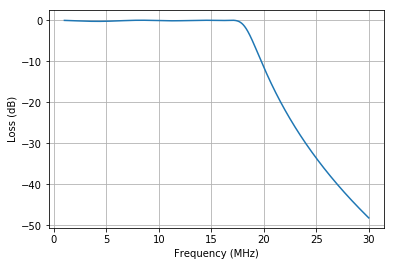In :
import numpy as np
import matplotlib.pyplot as plt

In :
uH = 1e-6
mH = 1e-3

pF = 1e-12
nF = 1e-9
uF = 1e-6

MHz = 1e6
kHz = 1e3

In :
class Port():
def z(self, f): return 1 / self.y(f)
def y(self, f): return 1 / self.z(f)

class Component(Port):
def __init__(self):
self.val = None

class Res(Component):
def __init__(self, ohms):
self.val = ohms
def z(self, f):
return self.val * np.ones_like(f)

class Coil(Component):
def __init__(self, henries):
self.val = henries
def z(self, f):
return 2 * np.pi * f * self.val * 1j

class Cap(Component):
def __init__(self, farads):
self.val = farads
def y(self, f):
return 2 * np.pi * f * self.val * 1j

class Series(Port):
def __init__(self, *elems):
self.elems = elems
def z(self, f):
return sum([port.z(f) for port in self.elems])

class Parallel(Port):
def __init__(self, *elems):
self.elems = elems
def y(self, f):
return sum([port.y(f) for port in self.elems])

In :
# This be the impedance, purely reactive.
Cap(0.1 * uF).z(10 * MHz)

Out:
-0.15915494309189535j
In :
# And this be the admittance, purely susceptant.
Cap(0.1 * uF).y(10 * MHz)

Out:
6.283185307179586j
In :
def Tee(p1, p2, p3, load):
return Series(p1, Parallel(p2, Series(p3, load)))

L1 = L3 = Coil(5.5 * uH)
L2 = Coil(2.6 * uH)
C7 = C9 = Cap(680 * pF)
C8 = Cap(1500 * pF)

bpf_1p8_4 = Tee(p1=Series(C7, L1), p2=Parallel(C8, L2), p3=Series(C9, L3), load=Res(50))

L4 = L6 = Coil(2.0 * uH)
L5 = Coil(0.46 * uH)
C11 = C13 = Cap(390 * pF)
C12 = Cap(1500 * pF)

bpf_4_8 = Tee(p1=Series(C11, L4), p2=Parallel(C12, L5), p3=Series(C13, L6), load=Res(50))

L7 = L9 = Coil(1 * uH)
L8 = Coil(0.27 * uH)
C14 = C16 = Cap(180 * pF)
C15 = Cap(680 * pF)

bpf_8_16 = Tee(p1=Series(C14, L7), p2=Parallel(C15, L8), p3=Series(C16, L9), load=Res(50))

L10 = L12 = Coil(0.46 * uH)
L11 = Coil(0.13 * uH)
C17 = C19 = Cap(100 * pF)
C18 = Cap(390 * pF)

bpf_16_30 = Tee(p1=Series(C17, L10), p2=Parallel(C18, L11), p3=Series(C19, L12), load=Res(50))

In :
def fplot(filt, source, load, f_start=1*MHz, f_end=30*MHz, f_step=1*kHz):
"""Filter response plot for filter fed by source.

The load is contained within the filter, the source is not.
"""

freq = np.arange(f_start, f_end, f_step)
z_f = filt.z(freq)
z_s = source.z(freq)
z_l = load.z(freq)

# We presently assume that the only resistive element of the filter
# is the load.  This is limiting.

filter_resp = z_f.real / abs(z_s + z_f)**2
load_resp =   z_l / (z_l + z_s)**2
response  = 10 * np.log10(filter_resp) - 10 * np.log10(load_resp)

fig, ax = plt.subplots()
ax.plot(freq / MHz, response)
ax.set_xlabel('Frequency (MHz)')
ax.set_ylabel('Loss (dB)')
ax.grid(True)

In :
fplot(bpf_1p8_4, source=Res(50), load=Res(50), f_start=500*kHz, f_end=60*MHz)
fplot(bpf_4_8, source=Res(50), load=Res(50), f_start=1.8*MHz, f_end=60*MHz)
fplot(bpf_8_16, source=Res(50), load=Res(50), f_start=1.8*MHz, f_end=60*MHz)
fplot(bpf_16_30, source=Res(50), load=Res(50), f_start=5*MHz, f_end=60*MHz)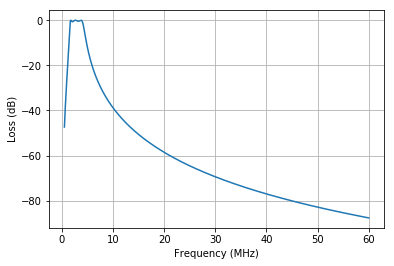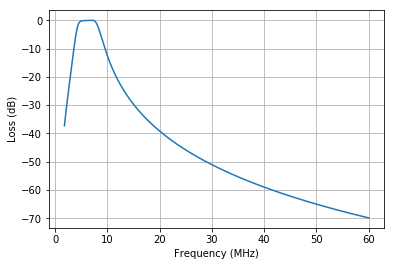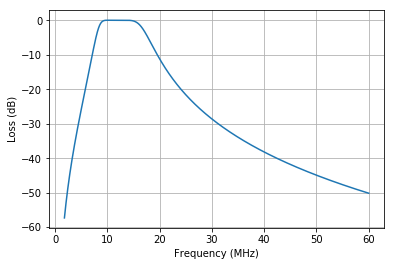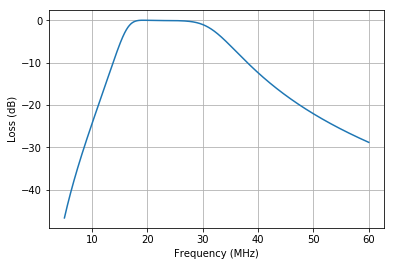In :
# The passband edge of bpf_8_16 is close to the 20 meter band.

# The actual filter shows only slight rolloff on 20 meters
# when fed with a perfectly-matched antenna or signal generator.

fplot(bpf_8_16, source=Res(50), load=Res(50), f_start=9.0*MHz, f_end=14.35*MHz)

# But the loss is more severe if fed with a high-impedance antenna,
# maybe a random wire.  We can try some reactive sources too--it might be different.
# Note also that this filter exhibits a transmatch-like gain at around 10 MHz,
# coupling the signal from the 300-ohm antenna to the load better than would
# happen by just connecting that antenna to the load directly.
fplot(bpf_8_16, source=Res(300), load=Res(50), f_start=9.0*MHz, f_end=14.35*MHz)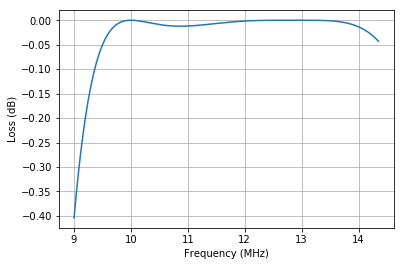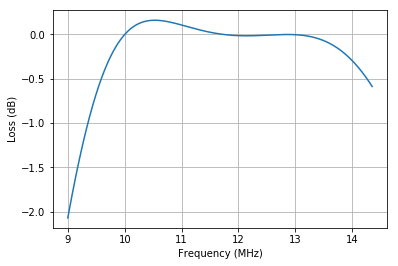In [ ]:


In :
def fr(L, C):
return 1 / (2 * np.pi * np.sqrt(L.val * C.val))

f1 = fr(L7, C14)
f2 = fr(L8, C15)
f3 = fr(L9, C16)

for f in [f1, f2, f3]:
print(f'{f / kHz :,.0f} kHz')

print(f'{L7.z(f1) :,.2f} ohms')
print(f'{L8.z(f2) :,.2f} ohms')
print(f'{L9.z(f3) :,.2f} ohms')

11,863 kHz
11,746 kHz
11,863 kHz
0.00+74.54j ohms
0.00+19.93j ohms
0.00+74.54j ohms

In :
def zplots(ckt, f_start=1*MHz, f_end=30*MHz, f_step=1*kHz):
f = np.arange(f_start, f_end, f_step)
z = ckt.z(f)

fig, mag = plt.subplots()
mag.semilogy(f, abs(z))
mag.set_xlabel('Frequency')
mag.set_ylabel('Z Magnitude')
mag.grid(True)

fig, phase = plt.subplots()
phase.plot(f, np.angle(z, deg=True))
phase.set_xlabel('Frequency')
phase.set_ylabel('Z Degrees')
phase.grid(True)

fig, res = plt.subplots()
res.plot(f, np.real(z))
res.set_xlabel('Frequency')
res.set_ylabel('Z Real')
res.grid(True)


In :
def capacitor(freq, ohms):
"Capacitor that has ohms reactance at freq"
return Cap(1 / (2 * np.pi * freq * ohms))

def inductor(freq, ohms):
"Inductor that has ohms reactance at afreq"
return Coil(ohms / (2 * np.pi * freq))

In :
# Ideal filter with exact components for 8 to 16 MHz
R_out = Res(50)

C_12_75 = capacitor(12 * MHz, ohms=75)
L_12_75 = inductor(12 * MHz, ohms=75)

C_12_20 = capacitor(12 * MHz, ohms=20)
L_12_20 = inductor(12 * MHz, ohms=20)

bpf_8_16_ideal = Tee(p1=Series(C_12_75, L_12_75),
p2=Parallel(C_12_20, L_12_20),
p3=Series(C_12_75, L_12_75),
load=Res(50))

zplots(bpf_8_16_ideal)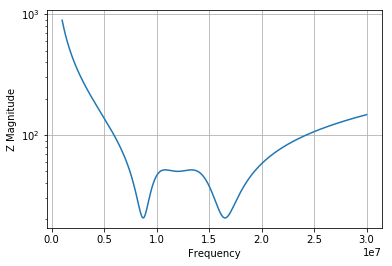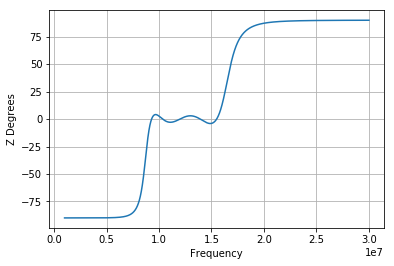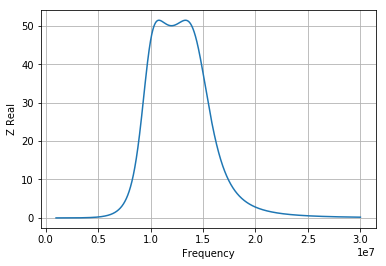In :
# Component values for this ideal filter.
print(C_12_75.val / pF, "pF")
print(L_12_75.val / uH, "uH")
print("")
print(C_12_20.val / pF, "pF")
print(L_12_20.val / uH, "uH")

176.83882565766146 pF
0.994718394324346 uH

663.1455962162306 pF
0.26525823848649227 uH

In :
zplots(bpf_8_16)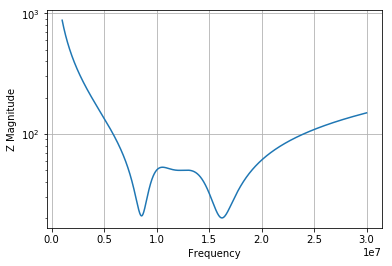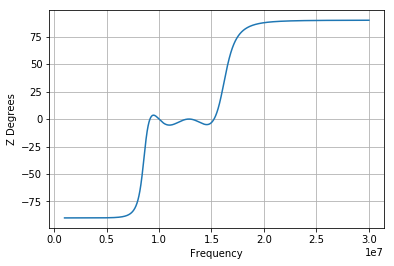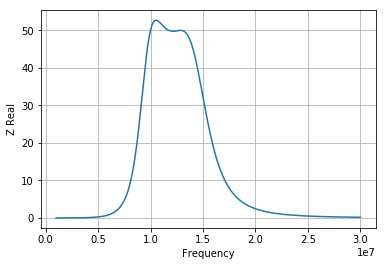In [ ]:


In :
def Pi(p1, p2, p3, load):
return Parallel(p1, Series(p2, Parallel(p3, load)))

def Attenuator(load):
return Pi(p1=Res(75), p2=Res(120), p3=Res(75), load=load)

In [ ]:


In :
# 8640B filters

# 8-16 MHz Low Band
C24 = Cap(360 * pF)
L10 = Coil(0.924 * uH)
C25 = Cap(640 * pF)
L11 = Coil(1.0 * uH)
C26 = Cap(640 * pF)
L12 = Coil(0.924 * uH)
C27 = Cap(390 * pF)

for component in [C24, L10, C25, L11, C26, L12, C27]:
print(component.z( (8 + 11.313)/2 * MHz))

-45.782329430347815j
56.06238692095243j
-25.752560304570647j
60.67357891877968j
-25.752560304570647j
56.06238692095243j
-42.260611781859524j

In :
def Pi_3_Section(p1, p2, p3, p4, p5, p6, p7, load):
return Parallel(p1, Series(p2, Parallel(p3, Series(p4, Parallel(p5, Series(p6, Parallel(p7, load)))))))

rsys = 50
R_out = Res(rsys)

LPF_8_16_LO = Pi_3_Section(C24, L10, C25, L11, C26, L12, C27, load=R_out)

fplot(LPF_8_16_LO, Res(rsys), Res(rsys))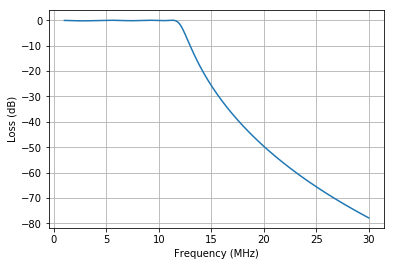In :
zplots(LPF_8_16_LO)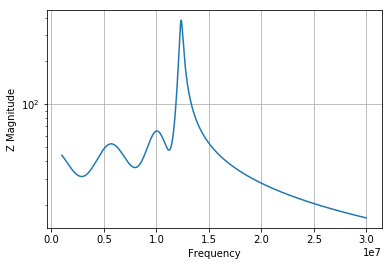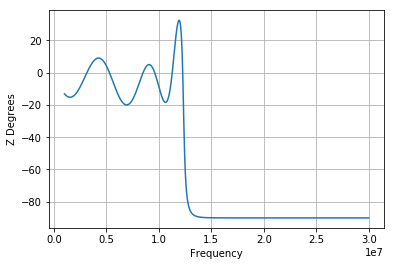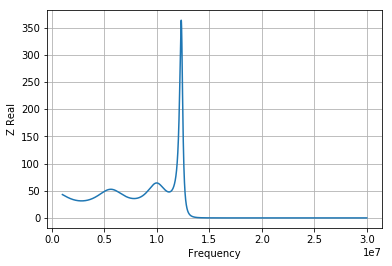In :
# 8-16 MHz High Band

C28 = Cap(240 * pF)
L13 = Coil(0.600 * uH)
C29 = Cap(430 * pF)
L14 = Coil(0.646 * uH)
C30 = Cap(430 * pF)
L15 = Coil(0.600 * uH)
C31 = Cap(240 * pF)

LPF_8_16_HI = Pi_3_Section(C28, L13, C29, L14, C30, L15, C31, load=R_out)

fplot(LPF_8_16_HI, Res(rsys), Res(rsys))